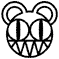Zero

Zero is the smallest number there is (even smaller than one) aside from creating an annoying footnote when you make the claim you can divide anything by any number zero is amongst the most useless number's in existence. It has no value at all literally. Unless you place another number or numbers in front of it, except of course another zero. Then it has value.

History

Zero was invented by the Babylonians, they realized that if they ate 2 apples after having 2 apples originally they would no longer have any apples left. They called this result "hunger". As the issue started applying to things other than food, such as limbs and or trees that called the result "zero" it was to be represented with an unhappy face at first, but as zero started cropping up in places such as having "zero diseases" or "zero dead villagers" they wanted to use a happy face. To compromise the face was left out completely and simply represented with a circle.

Simple maths!

Doing maths with zero takes the easiest and hardest form of mathematics. For instance addition with zero is easy, as the result is always what you are adding the zero to, and unlike most things in maths there are no exceptions!

Subtraction with zero is also fairly simple, it is also the same as what you started with. This however does not mean addition is equal to subtraction because they are opposites like the cake and the lie.

Multiplication with zero is actually by fair the easiest, because the result is in fact always zero again. Thank about it you can't have zero lots of something and end up with having something. Only in cases where the law of conservation of mass is not taken into account can the result be something other than zero, even then it's kinda shifty because my calculator says so.

Division with zero is impossible. You cannot divide by zero, not even with another zero. The reason is you cannot split something amongst nothing without a remainder equal to what you started with. Dividing by zero has uses however, such as that used in proving 1 = 2 or the creation of blackholes in un-convenient locations.This page has received the blessing of The Great Gozimnot of Mathematics, and is therefore mathematically correct in all aspects.